# Intermediate Geometry : How to find the slope of parallel lines

## Example Questions

### Example Question #1 : How To Find The Slope Of Parallel Lines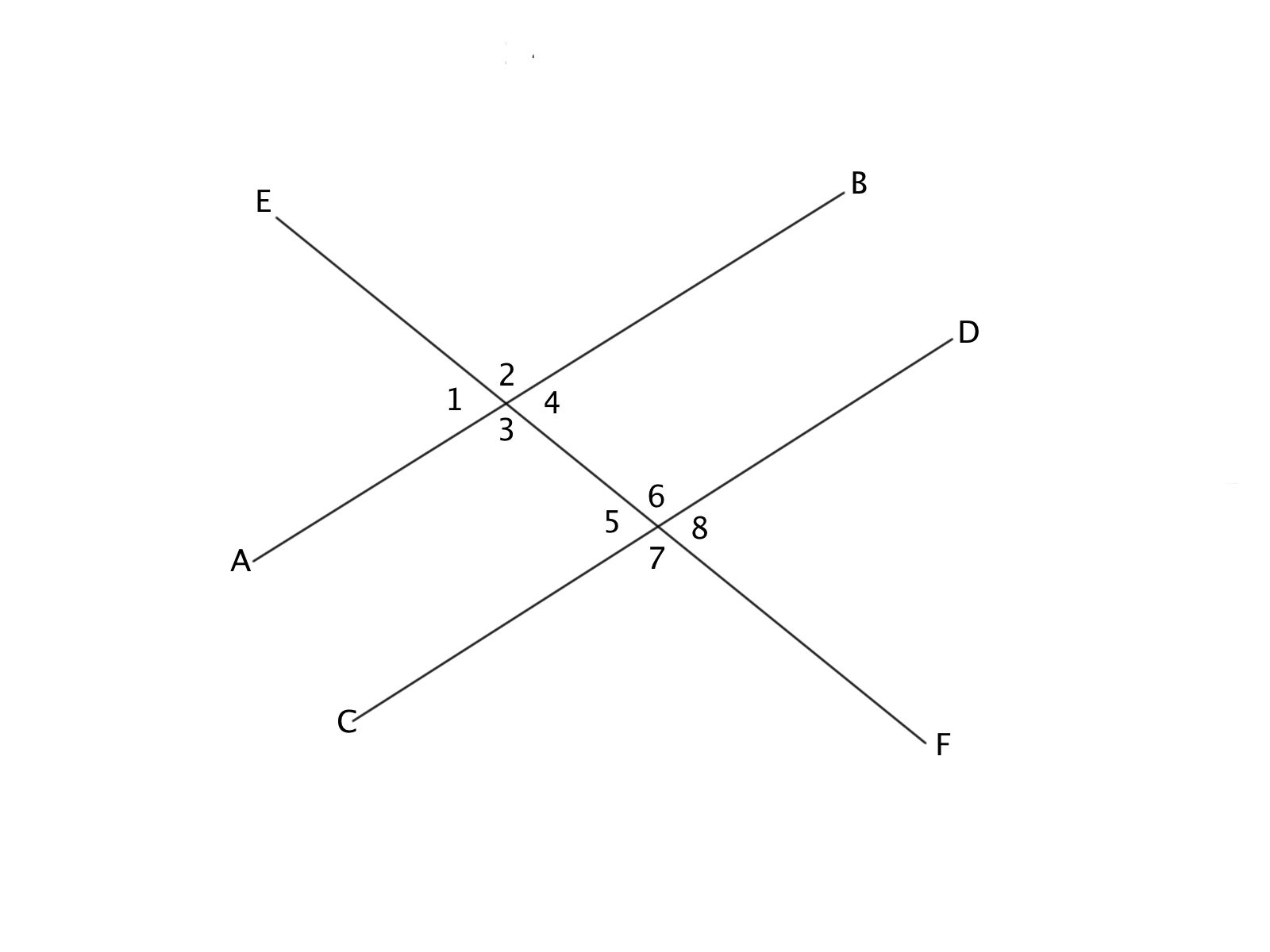If the slope of line AB is 3x, and Angle 1 and Angle 8 are congruent, what is the slope of line CD, and why?

(1/3)x, because of the Vertical Angle Theorem

3x, because of the Alternate Exterior Angle Theorem

(1/3)x, because of the Alternate Exterior Angle Theorem

3x, because of the Vertical Angle Theorem

3x, because of the Corresponding Angle Theorem

3x, because of the Vertical Angle Theorem

Explanation:

Angles 1 and 8 are a vertical pair. If these angles are congruent, it means that lines AB and CD are parallel based on the Vertical Angle Theorem. Parallel lines have the same slope, so the slope of CD is 3x.

### Example Question #2 : How To Find The Slope Of Parallel LinesThe slope of line CD is 4x, and Angle 1 and Angle 5 are congruent. What is the slope of line AB and why?

(1/4)x, because of the Corresponding Angle Theorem

There is not enough information to determine

4x, because of the Vertical Angle Theorem

4x, because of Alternate Interior Angles Theorem

4x, because of Corresponding Angles Theorem

4x, because of Corresponding Angles Theorem

Explanation:

Parallel lines have the same slope. If Angles 1 and 5 are congruent, then lines AB and CD have the same slope (4x) based on the Congruent Angles Theorem.

### Example Question #3 : How To Find The Slope Of Parallel Lines

Any line that is parallel to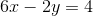must have a slope of what?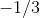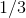Explanation:

Two lines are parallel if and only if they have the same slope. To find the slope, we must put the equation into slope-intercept form,, whereequals the slope of the line. First, we must subtract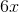from each side of the equation, giving us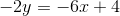. Next, we divide both sides by, giving us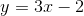. We can now see that the slope is.

### Example Question #4 : How To Find The Slope Of Parallel Lines

Suppose the equation of the first line is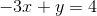.  What must be the value ofto make the second equation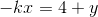parallel to the first line?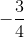Explanation:

Rewrite both equations so that they are in slope-intercept form,.

For the first equation: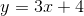The slope of the first line is.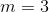Rewrite the second equation in slope-intercept form: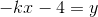The value of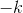must be equal to three to be parallel. Solve for.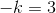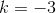### Example Question #5 : How To Find The Slope Of Parallel Lines

If the equation of one line is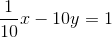, what must be the slope of another line so that both lines are parallel to each other?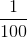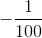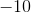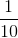Explanation:

Rewrite the equation of the first line in slope-intercept form,.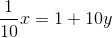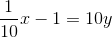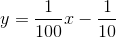The value of the slope,, can be seen as. For another line to be parallel to this line, their slopes must be the same.

### Example Question #6 : How To Find The Slope Of Parallel Lines

Find a line parallel to the line with the equation: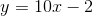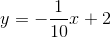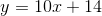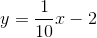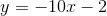Explanation:

For two lines to be parallel, they must have the same slope. For a line in, or slope intercept form,corresponds to the slope of the line.

For the given line,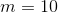. A line that is parallel must also then have the same slope.

Only the following line has the same slope:### Example Question #7 : How To Find The Slope Of Parallel Lines

Find a line parallel to the line with the equation: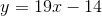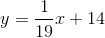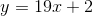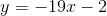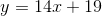Explanation:

For two lines to be parallel, they must have the same slope. For a line in, or slope intercept form,corresponds to the slope of the line.

For the given line,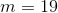. A line that is parallel must also then have the same slope.

Only the following line has the same slope:### Example Question #8 : How To Find The Slope Of Parallel Lines

Find a line parallel to the line with the equation: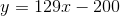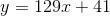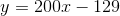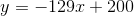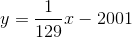Explanation:

For two lines to be parallel, they must have the same slope. For a line in, or slope intercept form,corresponds to the slope of the line.

For the given line,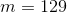. A line that is parallel must also then have the same slope.

Only the following line has the same slope:### Example Question #9 : How To Find The Slope Of Parallel Lines

Find a line parallel to the line with the equation: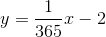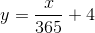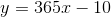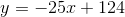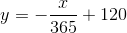Explanation:

For two lines to be parallel, they must have the same slope. For a line in, or slope intercept form,corresponds to the slope of the line.

For the given line,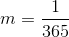. A line that is parallel must also then have the same slope.

Only the following line has the same slope: